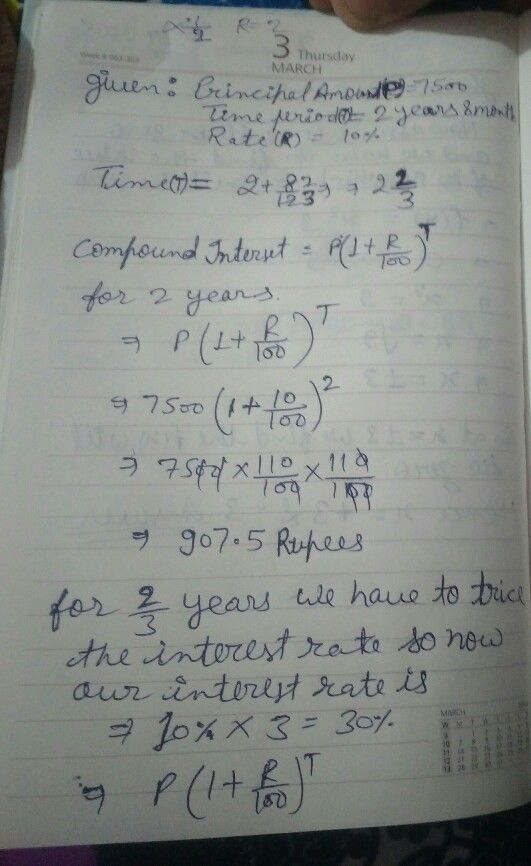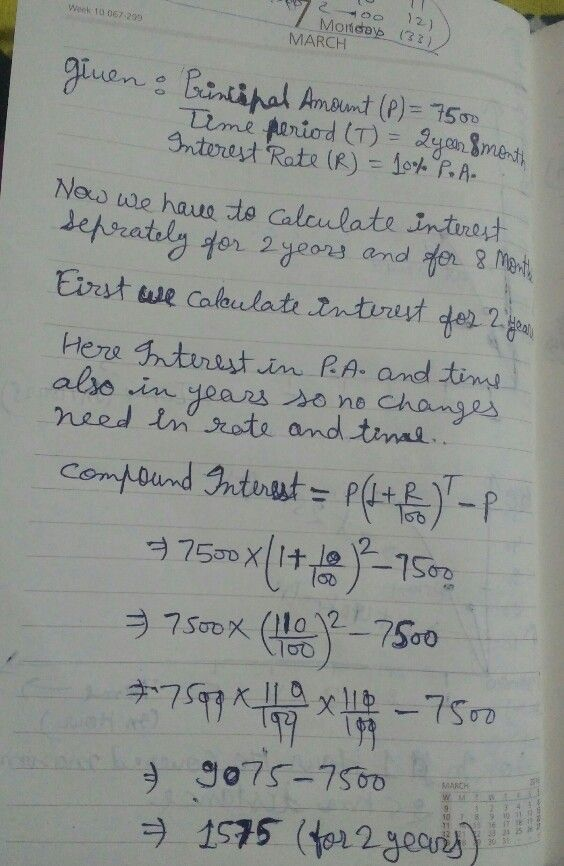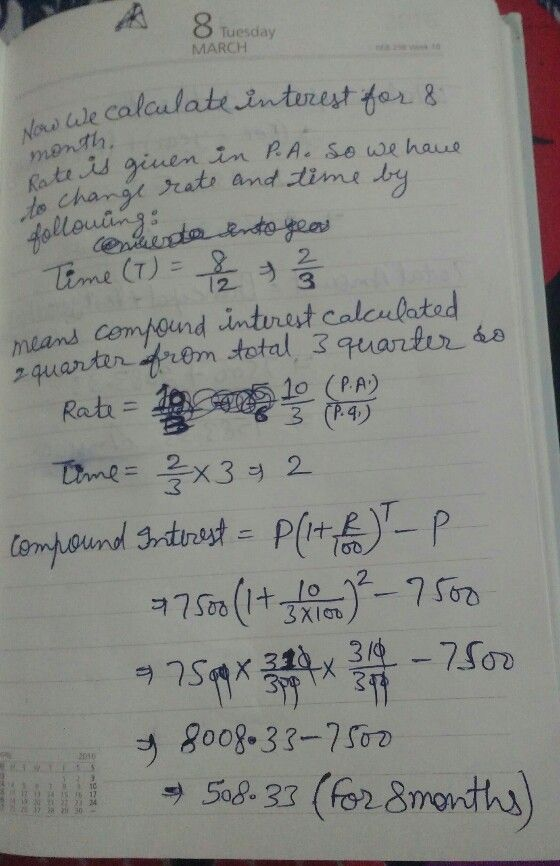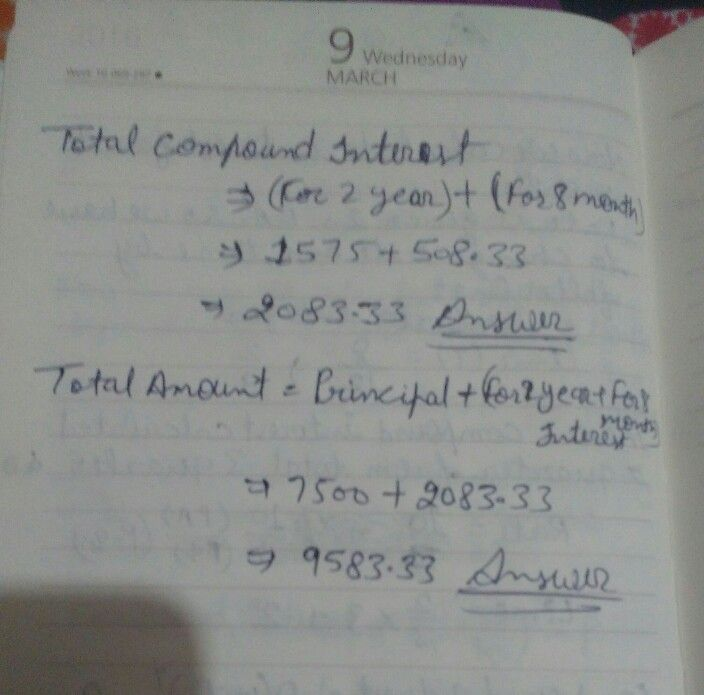Symbol
Problem$l0r$ the successive years being 8%, 9% and $10c$ compounded annually. 5. Find the amount and the compound interest $0D$ 7500 for $2$ years 8 months $a1$ $10$ p.a., compounded annually. 6. $11$ the simple interest on $a$ sum of money $lor$ $3\right)$ years at 8% per annum is $q$ $7500$ find the compound interest on the same sum, $10r$ the same period $at$ the same rate. $7.$ Calculate the amount and the compound interest $0D$ $16000$ for 1 year at $15%$ per annum,
Other
SolutionQanda teacher - worngStudent
Moreover 2/3 years to interest rates??Qanda teacher - worng